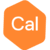# Simple new database examplePosts: 15edited February 23
Does there exist a simple example that creates a new database, adds a line, adds some channels, and puts some data in them? The example programs in GitHub include the following:
1 - NewGDB - Create a new DB, and do nothing with it - Don't set any symbols or add any data.
2 - Add chan - Open an existing DB, and edit some data in it.
3 - OMScript - Run a script

I don't find examples that bridge between step 1 and step 2, actually putting some structure into the new DB. I am trying to build an export facility from another program, to create and populate a new Geosoft DB.

I'd appreciate pointers to any examples.

-- Bob

• Hi Bob,

I don't think we have any .NET examples, we do have a collection of examples here https://github.com/GeosoftInc/gxc/tree/master/examples written in GXC.

Below is sample code that will create a line, add channels and add some data in those channels.
```var edb = CEDB.LoadNew(szName);
var db = edb.Lock();

var newLine = db.CreateSymb("L1", DB_SYMB_TYPEConstant.DB_SYMB_LINE, DB_OWNConstant.USER, DB_CATEGORY_LINEConstant.FLIGHT);

var xChan = db.CreateSymb("X", DB_SYMB_TYPEConstant.DB_SYMB_CHAN, DB_OWNConstant.USER, DB_CATEGORY_CHANConstant.DOUBLE);

var yChan = db.CreateSymb("Y", DB_SYMB_TYPEConstant.DB_SYMB_CHAN, DB_OWNConstant.USER, DB_CATEGORY_CHANConstant.DOUBLE);

var dataChan = db.CreateSymbEx("array_sample", DB_SYMB_TYPEConstant.DB_SYMB_CHAN, DB_OWNConstant.USER, DB_CATEGORY_CHANConstant.DOUBLE, 3);

using (CVV xVV = CVV.Create(GEO_VARConstant.GS_REAL, 0))
using (CVV yVV = CVV.Create(GEO_VARConstant.GS_REAL, 0))
using (CVA dataArrayVA = CVA.Create(GEO_VARConstant.GS_REAL, 5, 3))
{
for (int i = 0; i < 5; ++i)
{
xVV.SetReal(i, 10.0 * (i + 1));
yVV.SetReal(i, 1000.0 * (i + 1));
var rand = new Random();
for (int j = 0; j < 3; ++j)
{
dataArrayVA.SetReal(i, j, rand.NextDouble());
}
}

db.PutChanVV(newLine, xChan, xVV);
db.PutChanVV(newLine, yChan, yVV);
db.PutChanVA(newLine, dataChan, dataArrayVA);
}

db.SetXYZChan(DB_CHAN_SYMBOLConstant.DB_CHAN_X, "X");
db.SetXYZChan(DB_CHAN_SYMBOLConstant.DB_CHAN_Y, "Y");

if (db != null) db.UnLockAllSymb();
if (edb != null && edb.iIsLocked() == 1) edb.UnLock();

edb.GotoLine(newLine);
•Posts: 15